Test: Eigenvalues & Eigenvectors - 2

# Test: Eigenvalues & Eigenvectors - 2 - Mechanical Engineering

Test Description

## 25 Questions MCQ Test GATE Mechanical (ME) 2024 Mock Test Series - Test: Eigenvalues & Eigenvectors - 2

Test: Eigenvalues & Eigenvectors - 2 for Mechanical Engineering 2023 is part of GATE Mechanical (ME) 2024 Mock Test Series preparation. The Test: Eigenvalues & Eigenvectors - 2 questions and answers have been prepared according to the Mechanical Engineering exam syllabus.The Test: Eigenvalues & Eigenvectors - 2 MCQs are made for Mechanical Engineering 2023 Exam. Find important definitions, questions, notes, meanings, examples, exercises, MCQs and online tests for Test: Eigenvalues & Eigenvectors - 2 below.
Solutions of Test: Eigenvalues & Eigenvectors - 2 questions in English are available as part of our GATE Mechanical (ME) 2024 Mock Test Series for Mechanical Engineering & Test: Eigenvalues & Eigenvectors - 2 solutions in Hindi for GATE Mechanical (ME) 2024 Mock Test Series course. Download more important topics, notes, lectures and mock test series for Mechanical Engineering Exam by signing up for free. Attempt Test: Eigenvalues & Eigenvectors - 2 | 25 questions in 75 minutes | Mock test for Mechanical Engineering preparation | Free important questions MCQ to study GATE Mechanical (ME) 2024 Mock Test Series for Mechanical Engineering Exam | Download free PDF with solutions
 1 Crore+ students have signed up on EduRev. Have you?
Test: Eigenvalues & Eigenvectors - 2 - Question 1

### For the matrix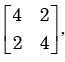the eigen value corresponding to the eigenvector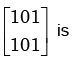Detailed Solution for Test: Eigenvalues & Eigenvectors - 2 - Question 1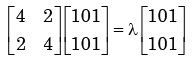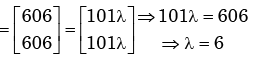*Multiple options can be correct
Test: Eigenvalues & Eigenvectors - 2 - Question 2

### The eigen values of the matrix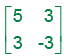Detailed Solution for Test: Eigenvalues & Eigenvectors - 2 - Question 2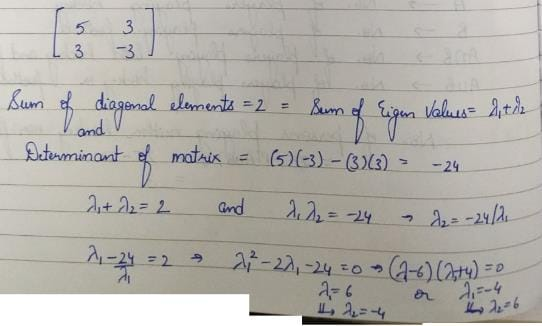Test: Eigenvalues & Eigenvectors - 2 - Question 3

### For the matrix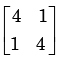the eigen value are

Detailed Solution for Test: Eigenvalues & Eigenvectors - 2 - Question 3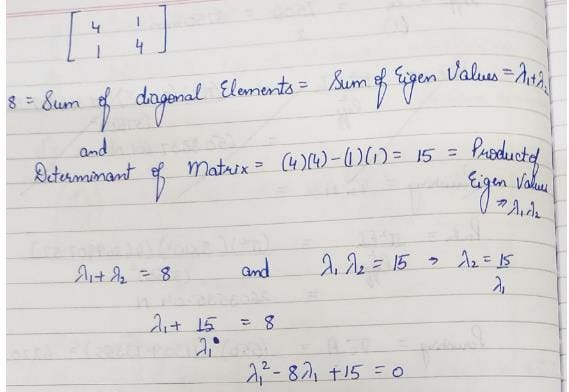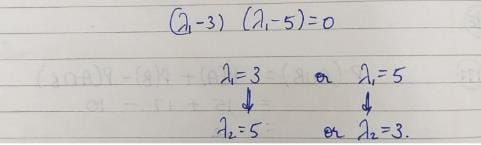Test: Eigenvalues & Eigenvectors - 2 - Question 4

For which value of x will the matrix given below become singular?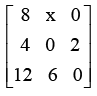Detailed Solution for Test: Eigenvalues & Eigenvectors - 2 - Question 4

Let the given matrix be A.  A is singular.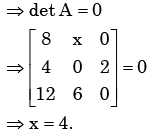Test: Eigenvalues & Eigenvectors - 2 - Question 5

If a square matrix A is real and symmetric, then the eigenvaluesn

Detailed Solution for Test: Eigenvalues & Eigenvectors - 2 - Question 5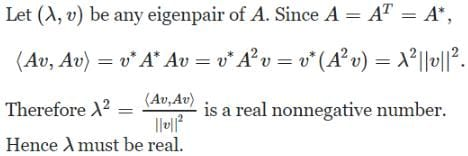Test: Eigenvalues & Eigenvectors - 2 - Question 6

The matrix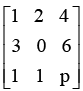has one eigenvalue equal to 3. The sum of the other two eigenvalues is

Detailed Solution for Test: Eigenvalues & Eigenvectors - 2 - Question 6

Let the given matrix be  A.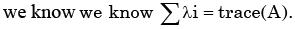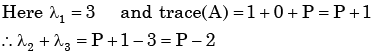Test: Eigenvalues & Eigenvectors - 2 - Question 7

For a matrix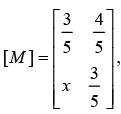the transpose of the matrix is equal to the inverse of the  matrix,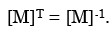The value of x is given by

Detailed Solution for Test: Eigenvalues & Eigenvectors - 2 - Question 7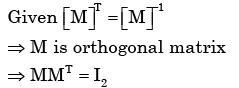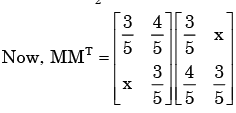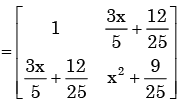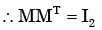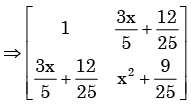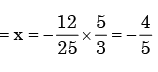Test: Eigenvalues & Eigenvectors - 2 - Question 8

The eigen values of the matrix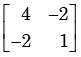Detailed Solution for Test: Eigenvalues & Eigenvectors - 2 - Question 8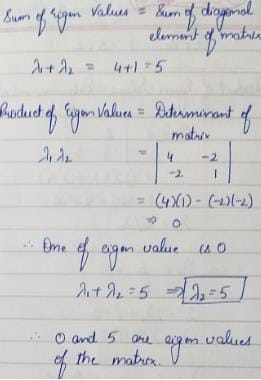Test: Eigenvalues & Eigenvectors - 2 - Question 9

For a given matrix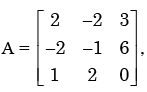one of the eigenvalues is 3. The other two eigenvalues are

Detailed Solution for Test: Eigenvalues & Eigenvectors - 2 - Question 9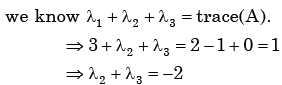Test: Eigenvalues & Eigenvectors - 2 - Question 10

The Eigen values of the matrix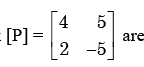Detailed Solution for Test: Eigenvalues & Eigenvectors - 2 - Question 10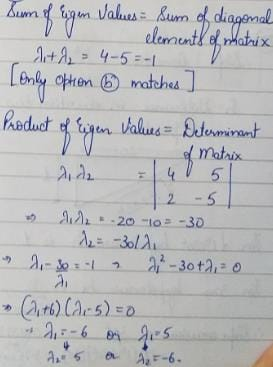Test: Eigenvalues & Eigenvectors - 2 - Question 11

In the matrix equation Px = q which of the following is a necessary condition for the existence of at least one solution for the unknown vector x:

Test: Eigenvalues & Eigenvectors - 2 - Question 12

If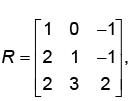then top row of R-1 is

Detailed Solution for Test: Eigenvalues & Eigenvectors - 2 - Question 12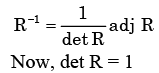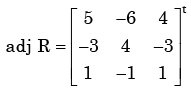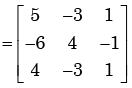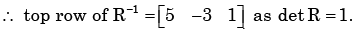Test: Eigenvalues & Eigenvectors - 2 - Question 13

Cayley - Hamiltion Theorem states that square matrix satisfies its own characteristic equation, Consider a matrix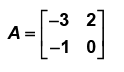A satisfies the relation

Detailed Solution for Test: Eigenvalues & Eigenvectors - 2 - Question 13

Characteristic equation of A is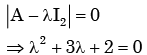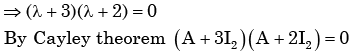Test: Eigenvalues & Eigenvectors - 2 - Question 14

The characteristic equation of a (3×3) matrix P is defined as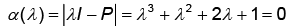If I denote identity matrix, then the inverse of matrix P will be

Detailed Solution for Test: Eigenvalues & Eigenvectors - 2 - Question 14

Given ch. equof A is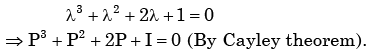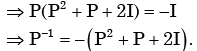Test: Eigenvalues & Eigenvectors - 2 - Question 15

Let P be a 2×2 real orthogonal matrix and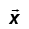s a real vector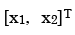with length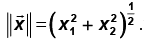Then which one of the following statements is correct?

Detailed Solution for Test: Eigenvalues & Eigenvectors - 2 - Question 15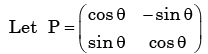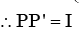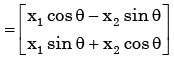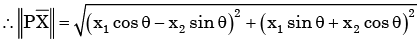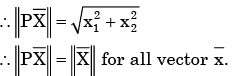Test: Eigenvalues & Eigenvectors - 2 - Question 16

An eigenvector of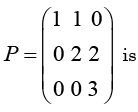Detailed Solution for Test: Eigenvalues & Eigenvectors - 2 - Question 16

Eigen values of P are 1,2,3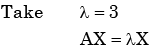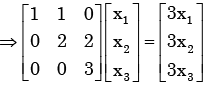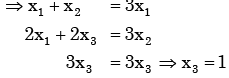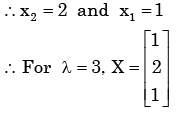Test: Eigenvalues & Eigenvectors - 2 - Question 17

Let A be an n × n real matrix such that A2 = I and y = be an n – dimensional vector.  Then the linear system of equations Ax = y has

Detailed Solution for Test: Eigenvalues & Eigenvectors - 2 - Question 17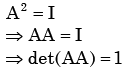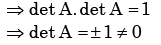By Cramer’s rule AX =y has unique solution.

Test: Eigenvalues & Eigenvectors - 2 - Question 18

A real n × n matrix A = {aij} is defined as follows:

aij = i = 0, if

i  = j, otherwise

The summation of all n eigen values of A is

Detailed Solution for Test: Eigenvalues & Eigenvectors - 2 - Question 18

It’s a diagonal marix diagonal contain’s  n elements 1,2,----,n.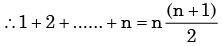As diagonal elements are eigen valves.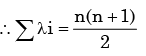Test: Eigenvalues & Eigenvectors - 2 - Question 19

The following system of equations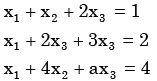has a unique solution. The only possible value(s) for a is/are

Detailed Solution for Test: Eigenvalues & Eigenvectors - 2 - Question 19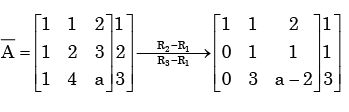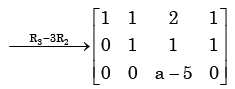System has unique Soln if  rank (A) = rank ( A ) = 3 . It is possible if a ≠ 5.

Test: Eigenvalues & Eigenvectors - 2 - Question 20

The eigenvalues of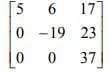are

Detailed Solution for Test: Eigenvalues & Eigenvectors - 2 - Question 20

The eigenvalues of an upper triangular matrix are simply the diagonal entries of the matrix. Hence 5, -19, and 37 are the eigenvalues of the matrix. Alternately, look at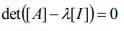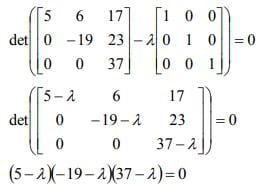Then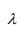= 5,-19,37 are the roots of the equation; and hence, the eigenvalues of [A].

Test: Eigenvalues & Eigenvectors - 2 - Question 21

The number of different n × n symmetric matrices with each element being either 0 or 1 is: (Note : power (2, x) is same as 2x)

Detailed Solution for Test: Eigenvalues & Eigenvectors - 2 - Question 21

In a symmetric matrix, the lower triangle must be the minor image of upper triangle using the diagonal as mirror. Diagonal elements may be anything. Therefore, when we are counting symmetric matrices we count how many ways are there to fill the upper triangle and diagonal elements. Since the first row has n elements, second (n – 1) elements, third row (n – 2) elements and so on upto last row, one element.  Total number of elements in diagonal + upper triangle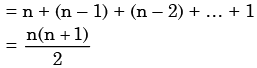Now, each one of these elements can be either 0 or 1. So that number of ways we can fill these elements is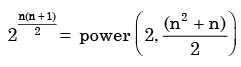Since there is no choice for lower triangle elements the answer is power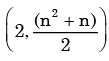which
is choice (c).

Test: Eigenvalues & Eigenvectors - 2 - Question 22

In an M × N matrix such that all non-zero entries are covered in a rows and b column. Then the maximum number of non-zero entries, such that no two are on the same row or column, is

Detailed Solution for Test: Eigenvalues & Eigenvectors - 2 - Question 22

Suppose a < b, for example let a = 3, b= 5, then we can put non-zero entries only in 3 rows and 5 columns. So suppose we put non-zero entries in any 3 rows in 3 different columns. Now we can’t put any other non-zero entry anywhere in matrix, because if we put it in some other row, then we will have 4 rows containing non-zeros, if we put it in one of those 3 rows, then we will have more than one non-zero entry in one row, which is not allowed.

So we can fill only “a” non-zero entries if a < b, similarly if b < a, we can put only “b” non-zero entries. So answer is ≤min(a,b), because whatever is less between a and b, we can put atmost that many non-zero entries.

Test: Eigenvalues & Eigenvectors - 2 - Question 23

Consider the following system of equation in three real variables x1, x2 and x3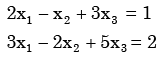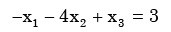This system of equations has

Detailed Solution for Test: Eigenvalues & Eigenvectors - 2 - Question 23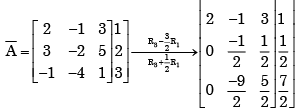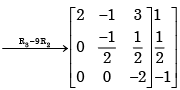∴ Rank (A)= Rank ( A ) = 3

Test: Eigenvalues & Eigenvectors - 2 - Question 24

How many of the following matrics have an eigenvalue 1?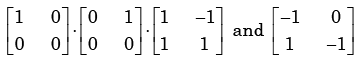Detailed Solution for Test: Eigenvalues & Eigenvectors - 2 - Question 24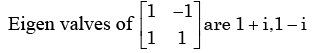Rest given matrix are triangular matrix. so diagonal elements are the eigen  values.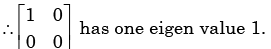Test: Eigenvalues & Eigenvectors - 2 - Question 25

What are the eigen values of the following 2 × 2 matrix?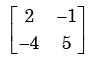Detailed Solution for Test: Eigenvalues & Eigenvectors - 2 - Question 25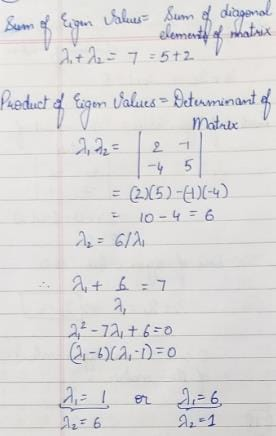## GATE Mechanical (ME) 2024 Mock Test Series

27 docs|243 tests
Information about Test: Eigenvalues & Eigenvectors - 2 Page
In this test you can find the Exam questions for Test: Eigenvalues & Eigenvectors - 2 solved & explained in the simplest way possible. Besides giving Questions and answers for Test: Eigenvalues & Eigenvectors - 2, EduRev gives you an ample number of Online tests for practice

## GATE Mechanical (ME) 2024 Mock Test Series

27 docs|243 tests